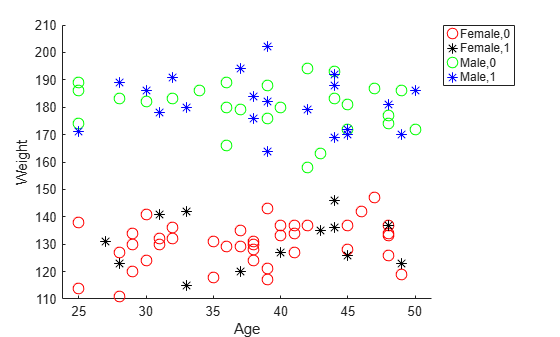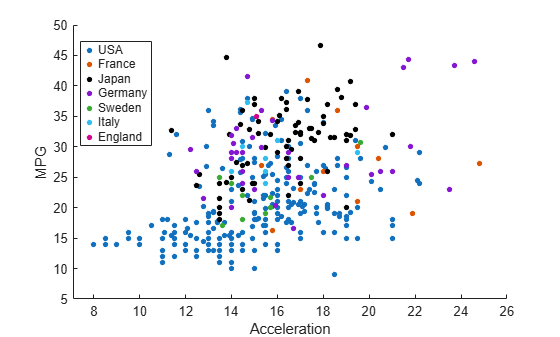Documentation

# gscatter

Scatter plot by group

## Syntax

``gscatter(x,y,g)``
``gscatter(x,y,g,clr,sym,siz)``
``gscatter(x,y,g,clr,sym,siz,doleg)``
``gscatter(x,y,g,clr,sym,siz,doleg,xnam,ynam)``
``h = gscatter(___)``

## Description

example

````gscatter(x,y,g)` creates a scatter plot of `x` and `y`, grouped by `g`. The inputs `x` and `y` are vectors of the same size.```

example

````gscatter(x,y,g,clr,sym,siz)` specifies the marker color `clr`, symbol `sym`, and size `siz` for each group.```
````gscatter(x,y,g,clr,sym,siz,doleg)` controls whether a legend is displayed on the graph. `gscatter` creates a legend by default.```

example

````gscatter(x,y,g,clr,sym,siz,doleg,xnam,ynam)` specifies the names to use for the x-axis and y-axis labels. If you do not provide `xnam` and `ynam`, and the `x` and `y` inputs are variables with names, then `gscatter` labels the axes with the variable names.```

example

````h = gscatter(___)` returns graphics handles corresponding to the groups in `g`, using any of the input arguments in the previous syntaxes.```

## Examples

collapse all

Load the `carsmall` data set.

`load carsmall`

Plot the `Displacement` values on the x-axis and the `Horsepower` values on the y-axis. `gscatter` uses the variable names as the default labels for the axes. Group the data points by `Model_Year`.

`gscatter(Displacement,Horsepower,Model_Year)`Load the `discrim` data set.

`load discrim`

The data set contains ratings of cities according to nine factors such as climate, housing, education, and health. The matrix `ratings` contains the ratings information.

Plot the relationship between the ratings for climate (first column) and housing (second column) grouped by city size in the matrix `group`. Choose different colors and plotting symbols for each group.

```gscatter(ratings(:,1),ratings(:,2),group,'br','xo') xlabel('climate') ylabel('housing')```Load the `hospital` data set.

`load hospital`

Plot the ages and weights of the hospital patients. Group the patients according to their gender and smoker status. Use the `o` symbol to represent nonsmokers and the `*` symbol to represent smokers.

```x = hospital.Age; y = hospital.Weight; g = {hospital.Sex,hospital.Smoker}; gscatter(x,y,g,'rkgb','o*',8,'on','Age','Weight') legend('Location','northeastoutside')```Load the `carbig` data set.

`load carbig`

Create a scatter plot comparing `Acceleration` to `MPG`. Group data points based on `Origin`.

`h = gscatter(Acceleration,MPG,Origin)`
```h = 7x1 Line array: Line (USA) Line (France) Line (Japan) Line (Germany) Line (Sweden) Line (Italy) Line (England) ```

Display the `Line` object corresponding to the group labeled `(Japan)`.

`jgroup = h(3)`
```jgroup = Line (Japan) with properties: Color: [0.2857 1 0] LineStyle: 'none' LineWidth: 0.5000 Marker: '.' MarkerSize: 15 MarkerFaceColor: 'none' XData: [1x79 double] YData: [1x79 double] ZData: [1x0 double] Show all properties ```

Change the marker color for the `Japan` group to black.

`jgroup.Color = 'k';`## Input Arguments

collapse all

x-axis values, specified as a numeric vector. `x` must have the same size as `y`.

Data Types: `single` | `double`

y-axis values, specified as a numeric vector. `y` must have the same size as `x`.

Data Types: `single` | `double`

Grouping variable, specified as a categorical vector, logical vector, numeric vector, character array, string array, or cell array of character vectors. Alternatively, `g` can be a cell array containing several grouping variables (such as ```{g1 g2 g3}```), in which case observations are in the same group if they have common values of all grouping variables. Points in the same group appear on the scatter plot with the same marker color, symbol, and size.

Example: `species`

Example: `{Cylinders,Origin}`

Data Types: `categorical` | `logical` | `single` | `double` | `char` | `string` | `cell`

Marker colors, specified as either a character vector or string scalar of colors recognized by the `plot` function or a matrix of RGB triplet values. Each RGB triplet is a three-element row vector whose elements specify the intensities of the red, green, and blue components of the color, respectively. Each intensity must be in the range [0,1].

This table lists the available color characters and their equivalent RGB triplet values.

Long NameShort NameRGB Triplet
Yellow`'y'``[1 1 0]`
Magenta`'m'``[1 0 1]`
Cyan`'c'``[0 1 1]`
Red`'r'``[1 0 0]`
Green`'g'``[0 1 0]`
Blue`'b'``[0 0 1]`
White`'w'``[1 1 1]`
Black`'k'``[0 0 0]`

If you do not specify enough values for all groups, then `gscatter` cycles through the specified values as needed.

Example: `'rgb'`

Example: `[0 0 1; 0 0 0]`

Data Types: `char` | `string` | `single` | `double`

Marker symbols, specified as a character vector or string scalar of symbols recognized by the `plot` function. This table lists the available marker symbols.

ValueDescription
`'o'`Circle
`'+'`Plus sign
`'*'`Asterisk
`'.'`Point
`'x'`Cross
`'s'`Square
`'d'`Diamond
`'^'`Upward-pointing triangle
`'v'`Downward-pointing triangle
`'>'`Right-pointing triangle
`'<'`Left-pointing triangle
`'p'`Five-pointed star (pentagram)
`'h'`Six-pointed star (hexagram)
`'none'`No markers

If you do not specify enough values for all groups, then `gscatter` cycles through the specified values as needed.

Example: `'o+*v'`

Data Types: `char` | `string`

Marker sizes, specified as a positive numeric vector in points. The default value is determined by the number of observations. If you do not specify enough values for all groups, then `gscatter` cycles through the specified values as needed.

Example: `[6 12]`

Data Types: `single` | `double`

Option to include a legend, specified as either `'on'` or `'off'`. By default, the legend is displayed on the graph.

x-axis label, specified as a character vector or string scalar.

Data Types: `char` | `string`

y-axis label, specified as a character vector or string scalar.

Data Types: `char` | `string`

## Output Arguments

collapse all

Graphics handles, returned as an array of `Line` objects. Each `Line` object corresponds to one of the groups in `g`. You can use dot notation to query and set properties of the line objects. For a list of `Line` object properties, see Line Properties.

Download ebook